# High School Math : Vector

## Example Questions

2 Next →

### Example Question #9 : Understanding Vector Calculations

Solve for vector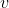given direction of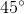and magnitude of.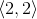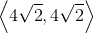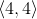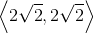Explanation:

To solve for a vector with the magnitude and direction given, we use the following formula: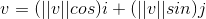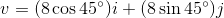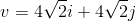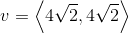### Example Question #10 : Understanding Vector Calculations

Given vector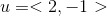and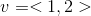, solve for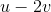.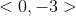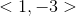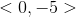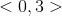Explanation: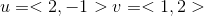To solve for, We need to multiplyinto vectorto find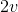; then we need to subtract thecomponents in the vector and the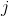components together: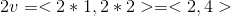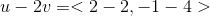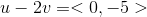### Example Question #21 : Calculus Ii — Integrals

Find the magnitude of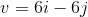.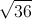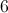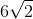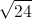Explanation:therefore the vector is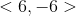To solve for the magnitude: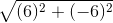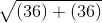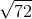### Example Question #22 : Calculus Ii — Integrals

Let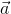and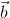be the following vectors: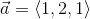and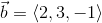. If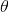is the acute angle between the vectors, then which of the following is equal to?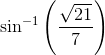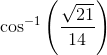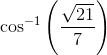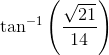Explanation:

The cosine of the acute angle between two vectors is given by the following formula: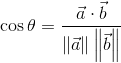, where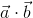represents the dot product of the two vectors,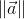is the magnitude of vector a, and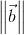is the magnitude of vector b.

First, we will need to compute the dot product of the two vectors. Let's say we have two general vectors in space (three dimensions),and. Let the components ofbe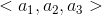and the components ofbe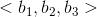. Then the dot productis defined as follows: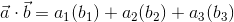.

Going back to the original problem, we can use this definition to find the dot product of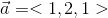and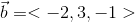.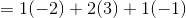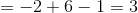The next two things we will need to compute areandLet the components of a general vectorbe. Thenis defined as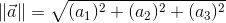.

Thus, ifand, then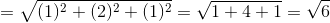and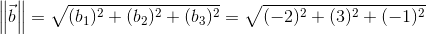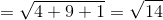.

Now, we put all of this information together to find the cosine of the angle between the two vectors.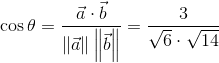We just need to simplify this.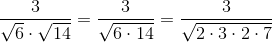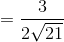.

In order to get it completely simplified, we have to rationalize the denominator by multiplying the numerator and denominator by the sqare root of 21.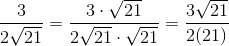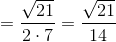.

We just have one more step. We need to solve for the value of the angle. In order to do this, we can take the inverse cosine of both sides of the equation.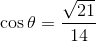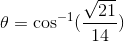.

The answer is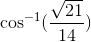.

2 Next →